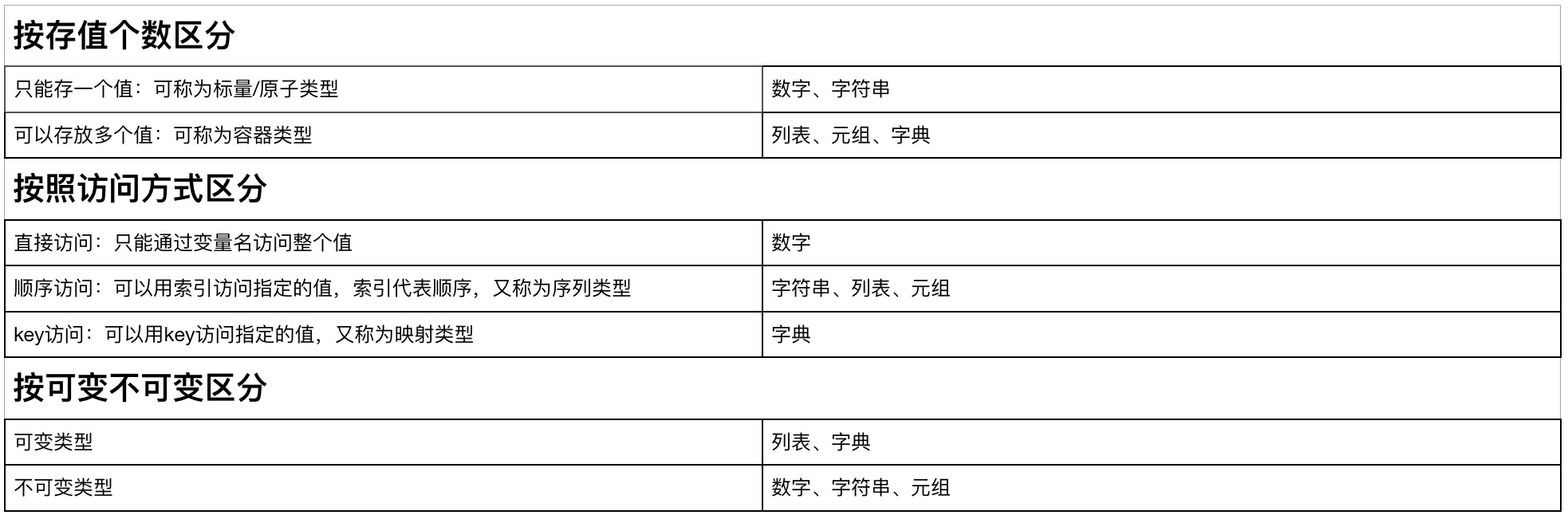# 元组  tuple

## 元组的类型总结：有序，不可变，存多个值

```t1 = ('a','b','c','a')  # t1 = tuple(('a','b','c'))
print(t1)
print(type(t1))

('a', 'b', 'c', 'a')
<class 'tuple'>```

# 常用方法

## 1、索引取值

```t1 = ('a','b','c','a')
print(t1)

a```

## 2、索引切片

```t1 = ('a','b','c','a')
print(t1[0:2])

('a', 'b')```

## 3、成员运算

```t1 = ('a','b','c','a')
print('a' in t1 )
print('a'not in t1 )

True
False```

## 4、len：获取当前元组中的元素个数

```t1 = ('a','b','c','a')
print(len(t1))

4```

## 5、count：统计当前元组中的某个元素的个数

```t1 = ('a','b','c','a')
print(t1.count('a'))

2```

## 6、index：获取当前元组的索引值，还可以指定查找范围

```t1 = ('a','b','c','a')
print(t1.index('b'))

1```

# 字典 dict

## 定义方式：通过大括号来存储数据，通过key:value来定义键值对数据，每个键值对中间通过逗号分隔

### key：一定是不可变类型        value：可以是任何类型

```# 1、
d1 = {'name':'egon','age':'84'}
print(d1)
# 2、
d2 = dict({'name':'egon','age':'84'})
print(d2)
# 3、
l1 = ['name','age']
l2 = ['egon','84']
z1 = zip(l1,l2)
print(dict(z1))

{'name': 'egon', 'age': '84'}
{'name': 'egon', 'age': '84'}
{'name': 'egon', 'age': '84'}```

## 1、按照key:value映射关系取值（可存可取可改）

```d1 = {'name':'egon','age':'84'}
print(d1['name'])
print(d1['age'])
d1['name'] = 'tank'
d1['gender'] = 'male'
print(d1)

egon
84
{'name': 'tank', 'age': '84', 'gender': 'male'}```

## 2、成员运算in，not in

```d1 = {'name':'egon','age':'84'}
print('egon' in d1)

False```

## 3、len:获取字典里键值对的个数

```d1 = {'name':'egon','age':'84'}
print(len(d1))

2```

## 4、get:获取指定key的值，如果不存在，默认返回None

```d1 = {'name':'egon','age':'84'}
print(d1.get('gender'))
# 可以通过第二个参数修改默认返回的内容
print(d1.get('gender','no'))

None
no```

## 5、keys、value、items：结合for循环使用

```d1 = {'name':'egon','age':'84'}
print(d1.keys())    # 返回所有的key
print(d1.values())  # 返回所有的value
print(d1.items())   # 返回所有的键值对，返回时列表元素
for keys in d1.keys():
print(keys)
for value in d1.values():
print(value)
for items in d1.items():
print(items)

dict_keys(['name', 'age'])
dict_values(['egon', '84'])
dict_items([('name', 'egon'), ('age', '84')])
name
age
egon
84
('name', 'egon')
('age', '84')```

## 6、pop：指定key进行删除，有返回值

```d1 = {'name':'egon','age':'84'}
d1.pop('name')
print(d1)

{'age': '84'}```

## 7、popitem：随机删除一组键值对，返删除键值对返回的是元组

```d1 = {'name':'egon','age':'84'}
d1.popitem()
print(d1)

{'name': 'egon'}```

## 8、update：用薪字典替换旧字典

```d1 = {'name':'egon','age':'84'}
d2 = {'1':'a'}
d1.update(d2)
print(d1)
d1.update({'name':'tank'})
print(d1)

{'name': 'egon', 'age': '84', '1': 'a'}
{'name': 'tank', 'age': '84', '1': 'a'}```

## 9、fromkeys：生成一个新字典，第一个参数他会以第一个参数的各个元素为key，以后第二个参数为值，组成一个新字典

```d1 = {'name':'egon','age':'84'}
print(dict.fromkeys([1,2,3],['ke','k1']))

{1: ['ke', 'k1'], 2: ['ke', 'k1'], 3: ['ke', 'k1']}```

## 10、setdefault：key不存在返回新增键值对的value，存在就返回存在的值

```d1 = {'name':'egon'}
print(d1.setdefault('name',1))
print(d1)

egon
{'name': 'egon'}```

# 集合

## 常用方法：

```# 集合中不可能出现两个相同的元素
python_student = {'egon', 'jason', 'tank', 'owen', 'egon'}
linux_student = {'frank', 'alex', 'egon'}
go_student = {'egon'}
print(python_student)
print(python_student | linux_student)
print(python_student & linux_student)
print(python_student - linux_student)
print(linux_student - python_student)
print(python_student ^ linux_student)
print(python_student > go_student)　　　　　　#判断父集
print(python_student < linux_student)　　　　#判断子集

{'jason', 'egon', 'tank', 'owen'}
{'tank', 'alex', 'owen', 'jason', 'egon', 'frank'}
{'egon'}
{'jason', 'tank', 'owen'}
{'frank', 'alex'}
{'alex', 'frank', 'tank', 'owen', 'jason'}
True
False```

# 类型总结：https://www.cnblogs.com/aheng/p/11806777.html

Python量化投资网携手4326手游为资深游戏玩家推荐：《《纯白魔女》：【转自投石姬】上级撒托古亚攻略

「点点赞赏，手留余香」

还没有人赞赏，快来当第一个赞赏的人吧！
Python
NumPy
0 条回复 A 作者 M 管理员
所有的伟大，都源于一个勇敢的开始！